# Introduction

The Fourier transform (FT) decomposes a signal into the frequencies that make it up.

…Let we have a signal 1, 2 and 3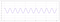Signal 1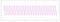Signal 2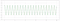Signal 3

y(t) = A. sin(2*pi*frequency*time + phase_difference)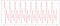Signal 1 + Signal2 + Signal3

# The mathematics behind Fourier Transform

The main idea behind Fourier transform is that :

Any continuous signal in the time domain can be represented uniquely and unambiguously by an infinite series of sinusoids.

# Discrete Fourier Transform

Any sampled signal of length N in the time domain can be represented uniquely and unambiguously by a finite series of sinusoids.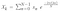Fourier Transform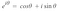Euler's Formula

So, If we plug Euler’s formula in Fourier Transform equation, we get

we also know that `cos(-θ) = cos(θ)` and `sin(-θ) = -sin(θ)` and if we use this in the above equation, the equation could be simplified as :

and we can actually break the above equation in two sums, such that :

Now, If we compare the above equation with the equation of a complex number which is `a + ib` then we get the corresponding value as :

So, now we can put these values in the equation of `f(t)` and get the result.

# Introduction

In the study of ancient astronomy:

… the epicycle is made to move in longitude in the order of the signs in a circle concentric with the Zodiac [i.e., from west to east], while the planet moves on the epicycle at a velocity which is the same as that of the anomaly; on that part of the epicycle farthest from the earth the motion is direct.

# Introduction

In the study of ancient astronomy:

… the epicycle is made to move in longitude in the order of the signs in a circle concentric with the Zodiac [i.e., from west to east], while the planet moves on the epicycle at a velocity which is the same as that of the anomaly; on that part of the epicycle farthest from the earth the motion is direct.

The main objective of this article is to explain how to create epicycles moving in a certain shape. For example, a motion could be as complicated as which is represented below:

import pygame as pg

from math import pi, sin, cos

white = (255, 255, 255)
gray = (100, 100, 100)
black = (0, 0, 0)

radius_scale = 100

def main():
time = 0
path = []

pg.init()
pg.font.init()
font = pg.font.SysFont(‘Consolas’, 24)

pg.display.set_caption(“Fourier”)

# CONFIG
width = 1000
height = 1000

start_x = 300
start_y = 500

wave_x = 700

terms = 2
time_step = 0.005
max_points = 2000

wave_choice = 1 # default to square (map at end of file)
###############

screen = pg.display.set_mode((width, height))

text = [“Select a wave:”,
“1: Square 2: Sawtooth 3: Triangle”,
“Up/Down: Number of terms Left/Right: Speed”]

running = True
pg.event.set_allowed([pg.QUIT, pg.KEYDOWN, pg.KEYUP])

while running:
for event in pg.event.get():
if event.type == pg.QUIT:
running = False
if event.type == pg.KEYDOWN:
keys = pg.key.get_pressed()
key = event.unicode
if keys[pg.K_ESCAPE]:
running = False
if keys[pg.K_UP]:
terms += 1
if keys[pg.K_DOWN] and terms > 1:
terms -= 1
if keys[pg.K_LEFT] and time_step > 0.001:
time_step -= 0.001
if keys[pg.K_RIGHT]:
time_step += 0.001
if key == ‘1’:
print(“switching to square wave”)
wave_choice = 1
if key == ‘2’:
print(“switching to sawtooth wave”)
wave_choice = 2
if key == ‘3’:
print(“switching to triangle wave”)
wave_choice = 3

x = start_x
y = start_y

# mark center point of circles
pg.draw.circle(screen, (255, 0, 0), (round(x), round(y)), 3)

circles = []
lines = [(start_x, start_y)]

for i in range(0, terms):
prev_x = x
prev_y = y

n, radius = waves[wave_choice](i)

# prevent “width greater than radius” exception
if abs(radius) < 1:
terms -= 1
break

x += radius * cos(n * time)
y += radius * sin(n * time)

circles.append(((round(prev_x), round(prev_y)), round(abs(radius))))
lines.append((x, y))

# draw circles first, then lines for clarity
print([circle for circle in circles])
for circle in circles:
pg.draw.circle(screen, gray, circle, circle, 1)

for i in range(0, len(lines) — 1):
pg.draw.line(screen, white, lines[i], lines[i + 1], 2)

# draw line from circles to wave
pg.draw.line(screen, gray, (x, y), (wave_x, y), 2)
path = add_point(path, [wave_x, y], time_step * 50, max_points)
draw_path(screen, path)

message_box(screen, font, text)
pg.display.update()
screen.fill(black)

time += time_step

def add_point(path, point, x_increment, max_points):
path = [[point + x_increment, point] for point in path]

path.insert(0, point)
return path[:max_points]

def draw_path(screen, path):
if len(path) > 1:
pg.draw.lines(screen, white, False, path, 2)

def message_box(root, font, text):
pos = 10
for x in range(len(text)):
rendered = font.render(text[x], 0, white)
root.blit(rendered, (10, pos))
pos += 30

def square_wave(i):
n = 2 * i + 1
radius = radius_scale * (4 / (n * pi))

return n, radius

def sawtooth_wave(i):
n = i + 1
radius = radius_scale * (2 * (-1 ** (n + 1)) / (n * pi))

return n, radius

def triangle_wave(i):
n = 2 * i + 1
# radius = radius_scale * 2 * (pi / 4) * (-1 ** i) * (n ** -2)
radius = (radius_scale * 3) * (4 * (1 — (-1 ** n))/ ((pi ** 2) * (n ** 2)))

return n, radius

waves = {1: square_wave,
2: sawtooth_wave,
3: triangle_wave}

if __name__ == “__main__”:

main()

curious...